Relativity and Cosmology Blog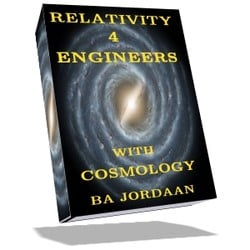# Relativity and Cosmology

This is a Blog on relativity and cosmology for engineers and the like. My website "Relativity-4-Engineers" has more in-depth stuff.

Comments/questions of a general nature should preferably be posted to the FAQ section of this Blog (http://cr4.globalspec.com/blogentry/316/Relativity-Cosmology-FAQ).

A complete index to the Relativity and Cosmology Blog can be viewed here: http://cr4.globalspec.com/blog/browse/22/Relativity-and-Cosmology"

Regards, Jorrie

 Previous in Blog: Spacetime Structure Next in Blog: Space-Propertime Structure and Acceleration II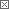# Space-Propertime Structure and Acceleration

Posted August 07, 2020 5:00 AM by Jorrie
Pathfinder Tags: Propertime spacetime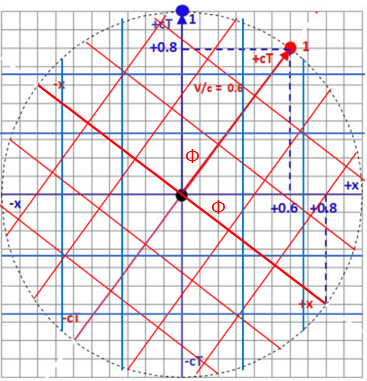I have introduced the idea of Epstein Space-Propertime Diagrams (ESPD) in my previous Blog post. To refresh, here is the ESPD's structural relationship between two relatively moving inertial frames. In this post I will extend the principle to accelerated motion.

Fig. 1 The case with no acceleration copied on the right

Three important features of the EPSD are:

1. Relative movement at a constant speed is equivalent to an Euclidean rotation around the origin by an angle: Φ = asin (v/c) e.g. the red Euclidean coordinate system relative to the blue one (where v/c is the relative velocity as a fraction of the speed of light). When v=c, the angle is pi/2, in contrast to the Minkowski diagram, where the angle would have been pi/4 (using atan Φ instead of asin Φ).

2. In flat spacetime (i.e. no gravity, spatial contraction or expansion), accelerated wordlines extend by a unity arc length per time unit, loosely stated as 'at the speed of light' around a 'curvature center'. This is a tad misleading, because it clashes with the standard meaning of speed and velocity, which are measured through space alone. The correct statement is that the magnitude of the 4-velocity (a vector through 3 spatial plus one time dimensions) remains constant.

3. This implies that while the acceleration lasts, the whole structure of the accelerated frame continually rotates around a shifting 'origin' over time. This is best pictured by a radius of curvature of individual worldlines around a shifting center of curvature. Fig. 2 depicts only one worldline going through the original origin for a small Δt.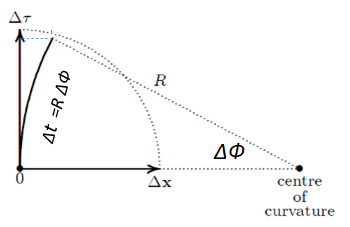Fig. 2: Initial acceleration from rest.

Note that in space-propertime diagramming, the coordinate time (Δt) is given by the arc, not by the vertical axis, which is propertime (Δtau) of course.

The initial center of curvature lies along the x-axis and the acceleration can be viewed as a centripetal force experienced by a hypothetical object moving at the speed of light around the center of curvature at radius R. This would cause centripetal acceleration of c/R2 in the +x direction.

Constant proper acceleration (as observed by in the accelerating frame) is equivalent to a continuous small rotation of the worldline around a shifting center of curvature, while the extension of the worldline is a unit four-vector per time unit . This gives the curved (parabolic) worldline segment Δt as indicated in Fig. 3.

Fig. 3 Continued constant proper accelerationIn most spacetime diagramming, geometric units like seconds and light-seconds, or year (yr) and lightyear (lyr) are used, so that c=1 lyr/yr and can be eliminated for simplicity.

The equation (lifted from the eBook) for the radius of curvature embodies the constant acceleration (x-dot-dot) and the relativistic factor cos Φ = √(1-v2). It shows how the relative speed can never exceed 1, because the radius of curvature will tend to infinity and the worldline to horizontal, i.e. the speed of light.

The constant proper acceleration is measured by an on-board accelerometer, so in the reference coordinate system, observed acceleration will tend to zero as Φ tends to pi/2.

This is in line with the standard relativity concept of momentum and energy tending to infinity as the relative speed tends to the speed of light. Not to distract from the natural flow of the idea, I will discuss energy and momentum in space-propertime at a later stage.

Here is a pseudo-code algorithm (lifted from the eBook) for calculating such an acceleration curve from the Fig. 3 point of view: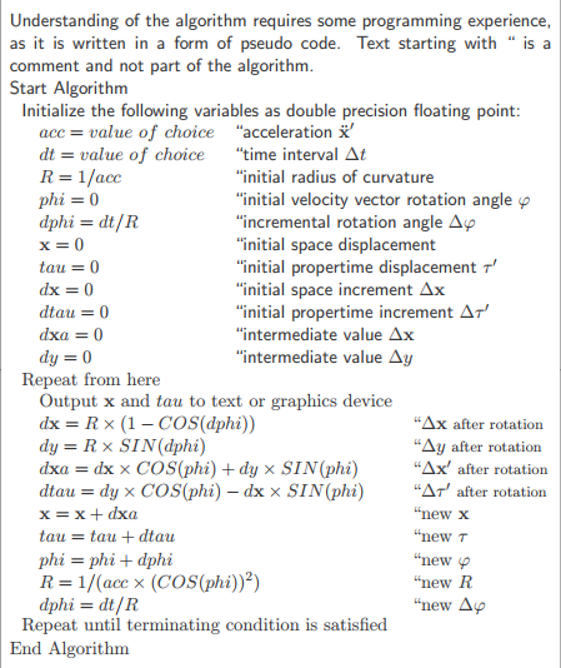It is largely self-explanatory, but just a few comments may be in order, I hope. The first 2 lines of code after the 'output' line simply rotates the present center of curvature to temporarily sit at a more convenient spot for calculation. The simpler calculation is performed and then the values are rotated back so that the new (x, tau) coordinate sits in the the correct place. One can't use precisely zero acceleration, but I stick in a value of 1E-12, being close enough to zero for all practical purposes.

I will now turn to a more practical-sounding example involving Alice and Bob, two space fairing friends, obtained from the algorithm above.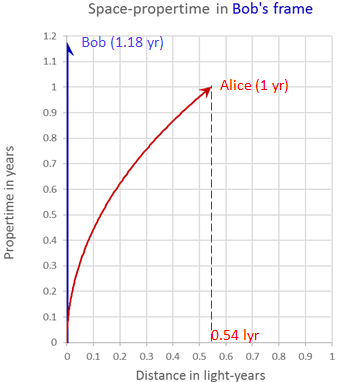On the right is a plot for Alice accelerating away from Bob at 1 lyr/y2, which is (interestingly) very close to 1g of proper acceleration, as we know it.

After one year on Alice's clock, with her accelerometer showing a constant 1g, she reaches a speed of 0.76c relative to the inertial Bob. Her distance from Bob is x=0.54 lyr, as shown. Bob's clock reads 1.18 yr at the same time, according to his definition of simultaneity (a horizontal line). Note that the blue vector and the red arc are of equal length.

The initial radius of curvature R = 1 lyr, but increases to R~2.4 lyr after the one year of onboard acceleration. In Bob's frame the acceleration drops from the initial 1g to 1/2.4~0.42g, due to the squared relativistic factor.

The Epstein diagrams are good for gaining insight in the workings, but relativists have derived exact solutions for calculating the curves analytically. The relevant equation are (using a for proper acceleration, t for coordinate time and T for propertime):

Edit: I have noticed that these equations were not simplified all the way, so I followed Einstein's advice...

x = (COSH(at)-1)/a , T=SINH(at)/a and v = TANH(at)

The hyperbolic trig functions show that the underlying spacetime structure is still hyperbolic in nature.

In a next Blog post I will use the analytical equations to plot more scenarios for Alice and Bob (and friends), which can be hugely informative.

-J

Interested in this topic? By joining CR4 you can "subscribe" to
Guru

Join Date: Jan 2006
Location: Tamworth, UK.
Posts: 1781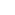#1

###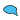Re: Space-Propertime Structure and Acceleration

08/08/2020 5:44 AM

Does this post support my theory that basic time units are changing - and thus causing an acceleration of mass in all directions at once ....the related force is something we measure/call/feel as gravity...?

The speed of light (assumed constant) - which is easier for me to understand as ratio of 'distance over time' - whereby the lengths we use to measure everything have also changed, and so has the 'size' of the Universe...and maybe no Red-Shift or Dark Matter ....

__________________
When arguing, remember mud-slinging = lost ground.
Guru

Join Date: Oct 2006
Location: 44.56024"N 15.307971E
Posts: 6931#2

###Re: Space-Propertime Structure and Acceleration

08/08/2020 6:10 AM

I was thinking along the same lines,and wondered if anyone else had considered it.Thanks!Acceleration,deceleration would be similar to changing the orbital radius of an object with mass.Add energy to increase,dissipate energy to decrease radius,which we perceive as inertia.__________________
"A man never stands so tall as when he stoops to help a child." "Never argue with a stupid person.They will drag you down to their level and beat you with experience"
Guru

Join Date: May 2006
Location: 34.02S, 22.82E
Posts: 3789#4

###Re: Space-Propertime Structure and Acceleration

08/08/2020 7:23 AM

Likewise, orbits happen in curved spacetime caused by gravity. The scheme discussed here is only valid in flat spacetime, no gravity. That said, it is possible to extend the space-propertime depiction to include gravity, but that adds the complication of another dimension, so that it is the '5-velocity' that equals the constant c.

I plan to move towards the gravity extension once we get far enough with the flat spacetime discussion.

__________________
"Perplexity is the beginning of knowledge." -- Kahlil Gibran
Guru

Join Date: Oct 2006
Location: 44.56024"N 15.307971E
Posts: 6931#5

###Re: Space-Propertime Structure and Acceleration

08/08/2020 9:49 AM

Can a straight line not be considered as a segment of a circle with an infinite radius?

If so,even "flat" spacetime has some finite curvature.

We would not be able to perceive it because we are in it and all of our measurements are affected by it.

Some one said if you ask a fish "How is the water?" he will reply "What is water?"

__________________
"A man never stands so tall as when he stoops to help a child." "Never argue with a stupid person.They will drag you down to their level and beat you with experience"
Guru

Join Date: May 2006
Location: 34.02S, 22.82E
Posts: 3789#6

###Re: Space-Propertime Structure and Acceleration

08/08/2020 2:13 PM

"If so, even "flat" spacetime has some finite curvature."

Sure, but you need appreciable spacetime curvature to consider orbits. Such a curvature is readily measurable in the Solar system. But that's off-topic here. This thread is about flat spacetime with acceleration caused by ordinary forces, like what a rocket would impart on a spaceship.

If you can hold your horses, there will be a future Blog on the way gravity influences the space-propertime structure.

__________________
"Perplexity is the beginning of knowledge." -- Kahlil Gibran
Guru

Join Date: May 2006
Location: 34.02S, 22.82E
Posts: 3789#3

###Re: Space-Propertime Structure and Acceleration

08/08/2020 7:05 AM

Nope, this has no bearing on cosmological physics. Here we talk about strictly local physics in flat spacetime. The expanding spacetime is not flat.

We have no evidence that basic time units are changing. I suppose one can concoct a theory where they do, but the end result has to be perfectly in alignment with Einstein's relativity, at least at the macro scales that we are talking about. Then Occon's razor will kick it out as an unnecessarily complex explanation.

__________________
"Perplexity is the beginning of knowledge." -- Kahlil Gibran